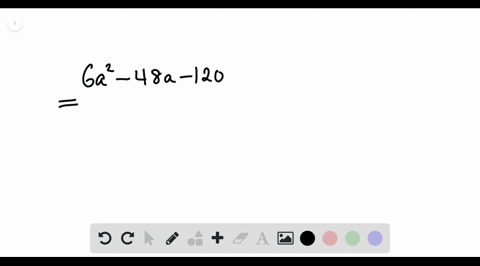Enroll in one of our FREE online STEM summer camps. Space is limited so join now!View Summer Courses### Factor each polynomial. If a polynomial cannot be…

01:00Other Schools

Need more help? Fill out this quick form to get professional live tutoring.

Get live tutoring
Problem 15

Factor each polynomial. If a polynomial cannot be factored, write prime. Factor out the greatest common factor as necessary.
$$21 m^{2}+13 m n+2 n^{2}$$

$21 m^{2}+13 m n+2 n^{2}=(7 m+2 n)(3 m+n)$

## Discussion

You must be signed in to discuss.

## Video Transcript

we're asked to factor this train a meal here, even though they're two variables here, you can treat it as if it's just a regular trying a meal with one variable. So this means we take our leading coefficient. 21 with are well, would have been our constant term of two, and we multiply them together. So this gives us 42. So now we will write down the factors of 42 and we will want to find a pair of factors that act together to be 13 so you can write them all down. But we know that six and seven multiply together to be 42 and it adds together to be 13. So the factors were looking for or six comma seven. So now what we do is we rewrite our try no meal, 21 m squared and then we split the middle term into two terms. So that would be six and then and seven and and then our last term of two and squared. So now we can look at this two terms at a time. So from the 1st 2 terms, the can factor ounce 3 a.m. and we're left with seven m plus two and and then from our 2nd 2 terms, we can't factor out an end. And we're left with seven m plus to end. So now we just have thes two terms. And as we can see, both of the those terms have the factor seven M plus to end. So that means you can factor that out and we're left with three. Um plus that.